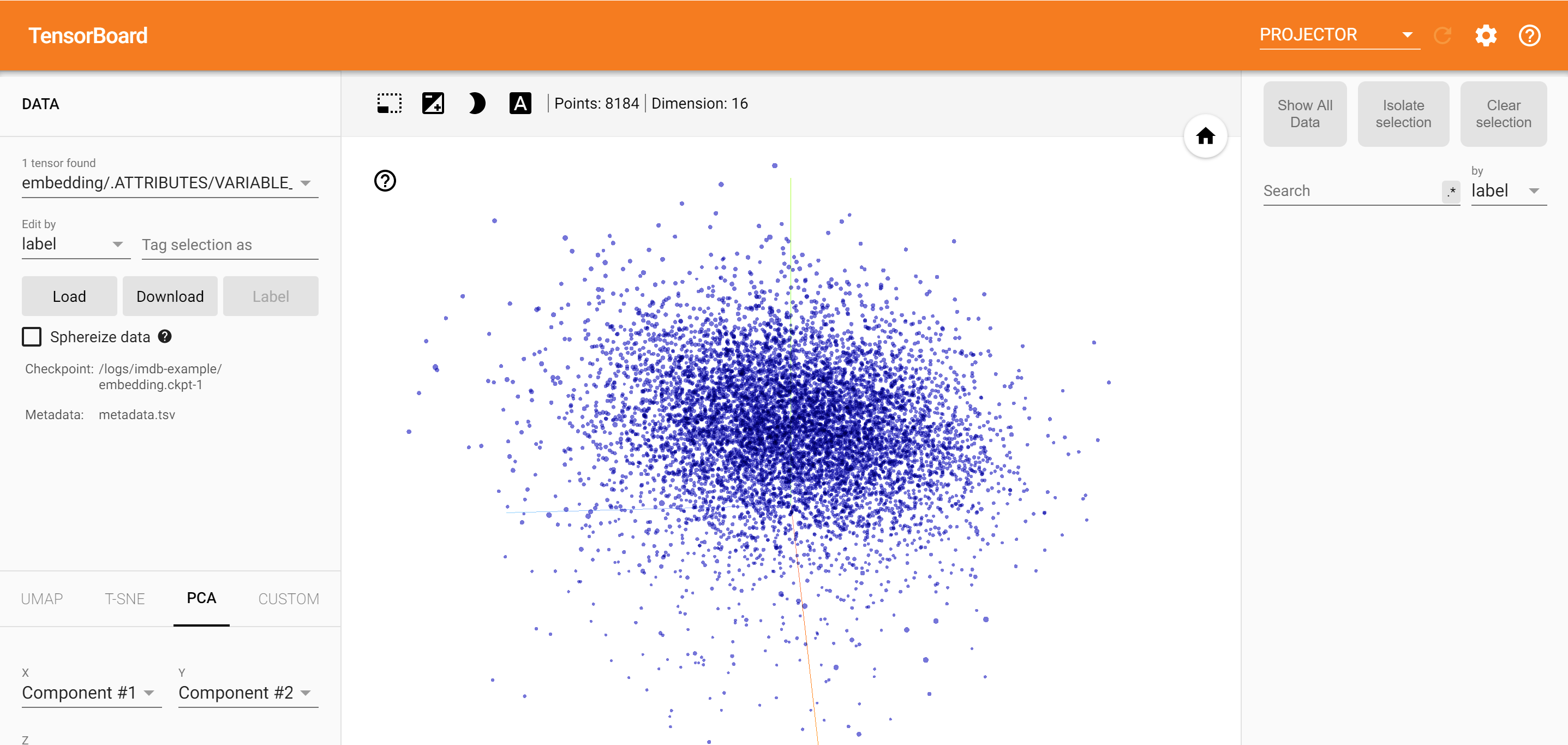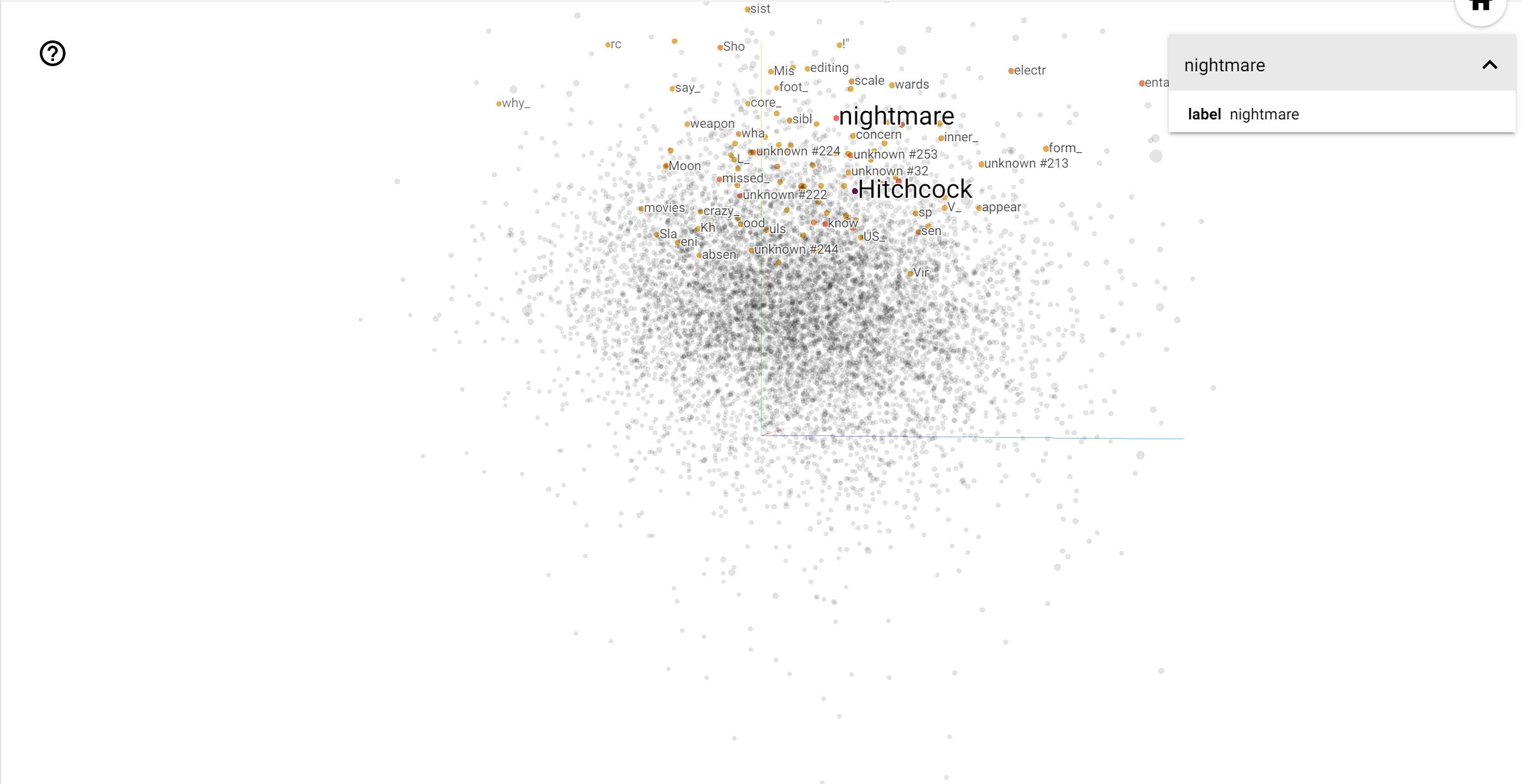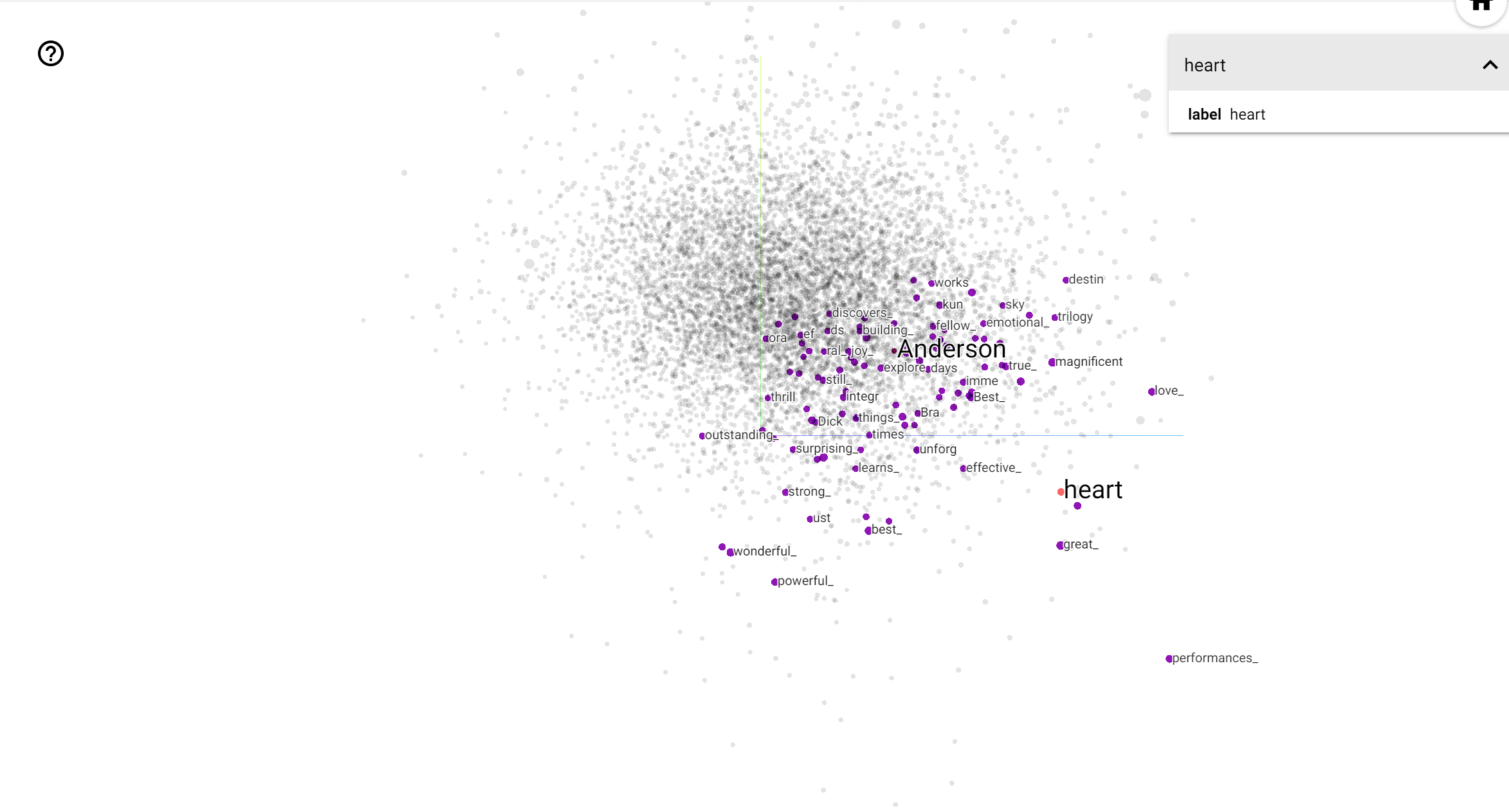# Visualizing Data using the Embedding Projector in TensorBoard

## Overview

Using the TensorBoard Embedding Projector, you can graphically represent high dimensional embeddings. This can be helpful in visualizing, examining, and understanding your embedding layers.In this tutorial, you will learn how visualize this type of trained layer.

## Setup

For this tutorial, we will be using TensorBoard to visualize an embedding layer generated for classifying movie review data.

``````try:
# %tensorflow_version only exists in Colab.
%tensorflow_version 2.x
except Exception:
pass

``````
``````import os
import tensorflow as tf
import tensorflow_datasets as tfds
from tensorboard.plugins import projector
``````

## IMDB Data

We will be using a dataset of 25,000 IMDB movie reviews, each of which has a sentiment label (positive/negative). Each review is preprocessed and encoded as a sequence of word indices (integers). For simplicity, words are indexed by overall frequency in the dataset, for instance the integer "3" encodes the 3rd most frequent word appearing in all reviews. This allows for quick filtering operations such as: "only consider the top 10,000 most common words, but eliminate the top 20 most common words".

As a convention, "0" does not stand for any specific word, but instead is used to encode any unknown word. Later in the tutorial, we will remove the row for "0" in the visualization.

``````(train_data, test_data), info = tfds.load(
"imdb_reviews/subwords8k",
split=(tfds.Split.TRAIN, tfds.Split.TEST),
with_info=True,
as_supervised=True,
)
encoder = info.features["text"].encoder

# Shuffle and pad the data.
)
)
train_batch, train_labels = next(iter(train_batches))
``````

# Keras Embedding Layer

A Keras Embedding Layer can be used to train an embedding for each word in your vocabulary. Each word (or sub-word in this case) will be associated with a 16-dimensional vector (or embedding) that will be trained by the model.

``````# Create an embedding layer.
embedding_dim = 16
embedding = tf.keras.layers.Embedding(encoder.vocab_size, embedding_dim)
# Configure the embedding layer as part of a keras model.
model = tf.keras.Sequential(
[
embedding, # The embedding layer should be the first layer in a model.
tf.keras.layers.GlobalAveragePooling1D(),
tf.keras.layers.Dense(16, activation="relu"),
tf.keras.layers.Dense(1),
]
)

# Compile model.
model.compile(
loss=tf.keras.losses.BinaryCrossentropy(from_logits=True),
metrics=["accuracy"],
)

# Train model for one epoch.
history = model.fit(
train_batches, epochs=1, validation_data=test_batches, validation_steps=20
)
``````
```2500/2500 [==============================] - 13s 5ms/step - loss: 0.5330 - accuracy: 0.6769 - val_loss: 0.4043 - val_accuracy: 0.7800
```

## Saving Data for TensorBoard

TensorBoard reads tensors and metadata from the logs of your tensorflow projects. The path to the log directory is specified with `log_dir` below. For this tutorial, we will be using `/logs/imdb-example/`.

In order to load the data into Tensorboard, we need to save a training checkpoint to that directory, along with metadata that allows for visualization of a specific layer of interest in the model.

``````# Set up a logs directory, so Tensorboard knows where to look for files.
log_dir='/logs/imdb-example/'
if not os.path.exists(log_dir):
os.makedirs(log_dir)

# Save Labels separately on a line-by-line manner.
with open(os.path.join(log_dir, 'metadata.tsv'), "w") as f:
for subwords in encoder.subwords:
f.write("{}\n".format(subwords))
# Fill in the rest of the labels with "unknown".
for unknown in range(1, encoder.vocab_size - len(encoder.subwords)):
f.write("unknown #{}\n".format(unknown))

# Save the weights we want to analyze as a variable. Note that the first
# value represents any unknown word, which is not in the metadata, here
# we will remove this value.
weights = tf.Variable(model.layers.get_weights()[1:])
# Create a checkpoint from embedding, the filename and key are the
# name of the tensor.
checkpoint = tf.train.Checkpoint(embedding=weights)
checkpoint.save(os.path.join(log_dir, "embedding.ckpt"))

# Set up config.
config = projector.ProjectorConfig()
# The name of the tensor will be suffixed by `/.ATTRIBUTES/VARIABLE_VALUE`.
embedding.tensor_name = "embedding/.ATTRIBUTES/VARIABLE_VALUE"
projector.visualize_embeddings(log_dir, config)
``````
``````# Now run tensorboard against on log data we just saved.
%tensorboard --logdir /logs/imdb-example/
``````## Analysis

The TensorBoard Projector is a great tool for interpreting and visualzing embedding. The dashboard allows users to search for specific terms, and highlights words that are adjacent to each other in the embedding (low-dimensional) space. From this example we can see that Wes Anderson and Alfred Hitchcock are both rather neutral terms, but that they are referenced in different contexts.In this space, Hitchcock is closer to words like `nightmare`, which is likely due to the fact that he is known as the "Master of Suspense", whereas Anderson is closer to the word `heart`, which is consistent with his relentlessly detailed and heartwarming style.[]
[]